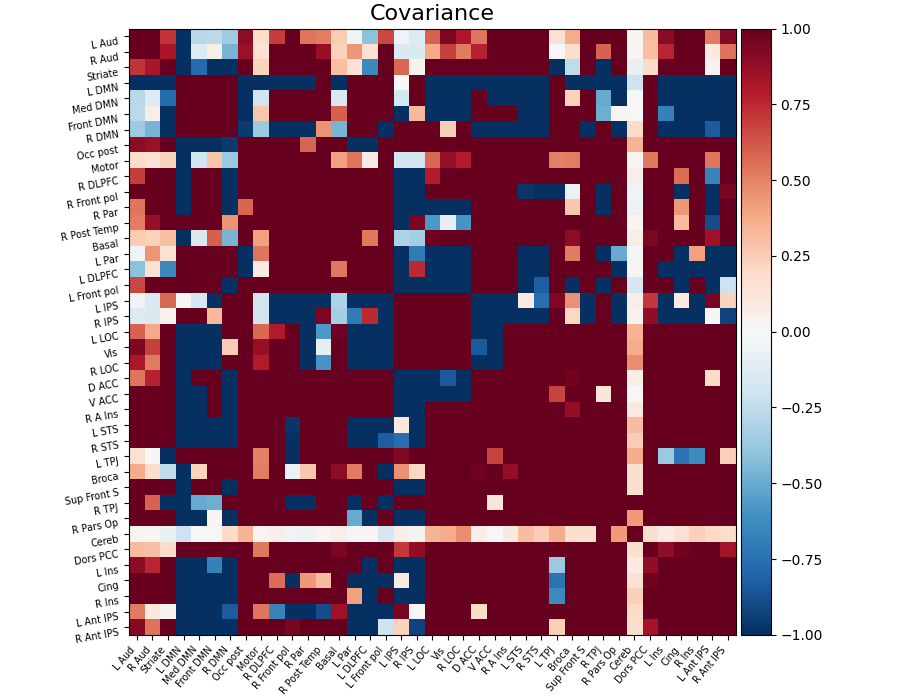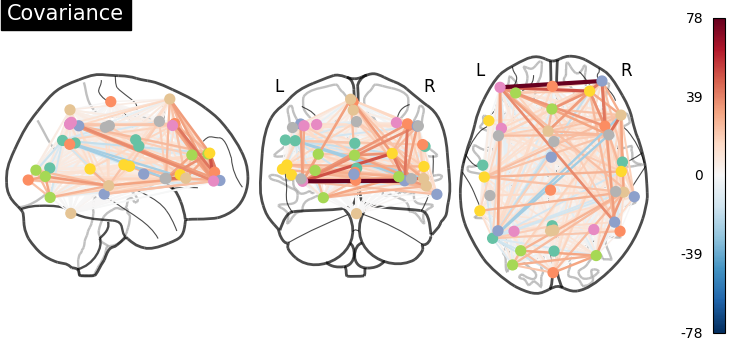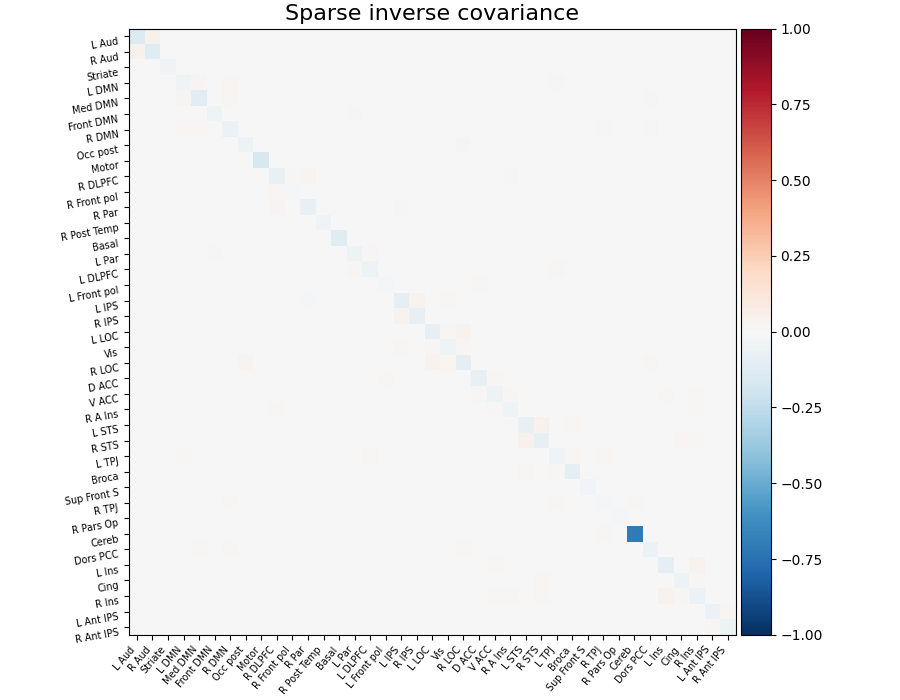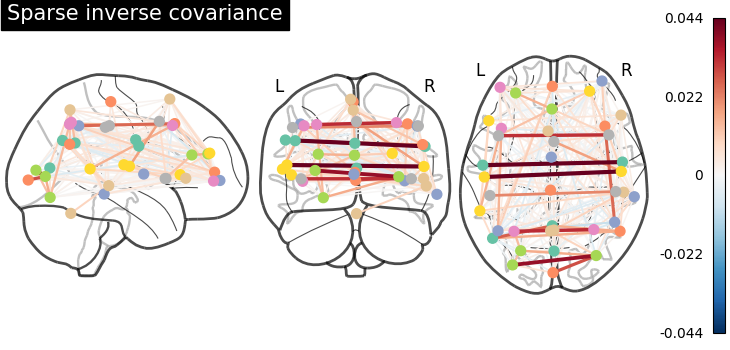# Computing a connectome with sparse inverse covariance#

This example constructs a functional connectome using the sparse inverse covariance.

We use the MSDL atlas of functional regions in movie watching, and the `nilearn.maskers.NiftiMapsMasker` to extract time series.

Note that the inverse covariance (or precision) contains values that can be linked to negated partial correlations, so we negated it for display.

As the MSDL atlas comes with (x, y, z) MNI coordinates for the different regions, we can visualize the matrix as a graph of interaction in a brain. To avoid having too dense a graph, we represent only the 20% edges with the highest values.

Note

If you are using Nilearn with a version older than `0.9.0`, then you should either upgrade your version or import maskers from the `input_data` module instead of the `maskers` module.

That is, you should manually replace in the following example all occurrences of:

```from nilearn.maskers import NiftiMasker
```

with:

```from nilearn.input_data import NiftiMasker
```

## Retrieve the atlas and the data#

```from nilearn import datasets

atlas = datasets.fetch_atlas_msdl()
atlas_filename = atlas["maps"]
labels = atlas["labels"]

data = datasets.fetch_development_fmri(n_subjects=1)

# print basic information on the dataset
print(f"First subject functional nifti images (4D) are at: {data.func}")
```
```First subject functional nifti images (4D) are at: /home/runner/work/nilearn/nilearn/nilearn_data/development_fmri/development_fmri/sub-pixar123_task-pixar_space-MNI152NLin2009cAsym_desc-preproc_bold.nii.gz
```

## Extract time series#

```from nilearn.maskers import NiftiMapsMasker

maps_img=atlas_filename,
standardize=True,
memory="nilearn_cache",
verbose=5,
)

```
```[NiftiMapsMasker.wrapped] loading regions from /home/runner/work/nilearn/nilearn/nilearn_data/msdl_atlas/MSDL_rois/msdl_rois.nii
Resampling maps
/usr/share/miniconda3/envs/testenv/lib/python3.9/site-packages/nilearn/_utils/cache_mixin.py:223: UserWarning:

memory_level is currently set to 0 but a Memory object has been provided. Setting memory_level to 1.

________________________________________resample_img cache loaded - 0.0s, 0.0min
__________________________________filter_and_extract cache loaded - 0.0s, 0.0min
```

## Compute the sparse inverse covariance#

```try:
from sklearn.covariance import GraphicalLassoCV
except ImportError:
# for Scitkit-Learn < v0.20.0
from sklearn.covariance import GraphLassoCV as GraphicalLassoCV

estimator = GraphicalLassoCV()
estimator.fit(time_series)
```
`GraphicalLassoCV()`
In a Jupyter environment, please rerun this cell to show the HTML representation or trust the notebook.

## Display the connectome matrix#

```from nilearn import plotting

# Display the covariance

# The covariance can be found at estimator.covariance_
plotting.plot_matrix(
estimator.covariance_,
labels=labels,
figure=(9, 7),
vmax=1,
vmin=-1,
title="Covariance",
)
``````<matplotlib.image.AxesImage object at 0x7f7ef851d4c0>
```

## And now display the corresponding graph#

```coords = atlas.region_coords

plotting.plot_connectome(estimator.covariance_, coords, title="Covariance")
``````<nilearn.plotting.displays._projectors.OrthoProjector object at 0x7f7ef0e6f640>
```

## Display the sparse inverse covariance#

we negate it to get partial correlations

```plotting.plot_matrix(
-estimator.precision_,
labels=labels,
figure=(9, 7),
vmax=1,
vmin=-1,
title="Sparse inverse covariance",
)
``````<matplotlib.image.AxesImage object at 0x7f7ee3ecd100>
```

## And now display the corresponding graph#

```plotting.plot_connectome(
-estimator.precision_, coords, title="Sparse inverse covariance"
)

plotting.show()
```## 3D visualization in a web browser#

An alternative to `nilearn.plotting.plot_connectome` is to use `nilearn.plotting.view_connectome` that gives more interactive visualizations in a web browser. See 3D Plots of connectomes for more details.

```view = plotting.view_connectome(-estimator.precision_, coords)

# In a Jupyter notebook, if ``view`` is the output of a cell, it will
# be displayed below the cell
view
```